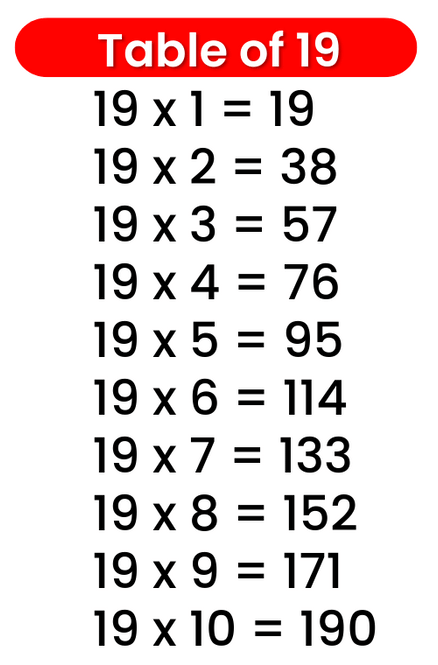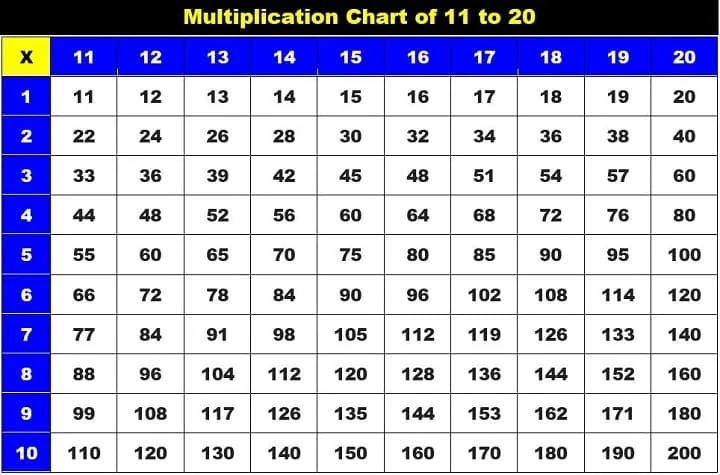# Times Table of 19, 19 ka table

## Times Table of 19, 19 ka table

Maths Multiplication Table of 19, Times Table of 19, 19 ka table, 19 ki Table Chart

19 ×‌ 1 = 19
19 ×‌ 2 = 38
19 ×‌ 3 = 57
19 ×‌ 4 = 76
19 ×‌ 5 = 95
19 ×‌ 6 = 114
19 ×‌ 7 = 133
19 ×‌ 8 = 152
19 ×‌ 9 = 171
19 ×‌ 10 = 190

## 19 ka table### Times Table of 19 chart